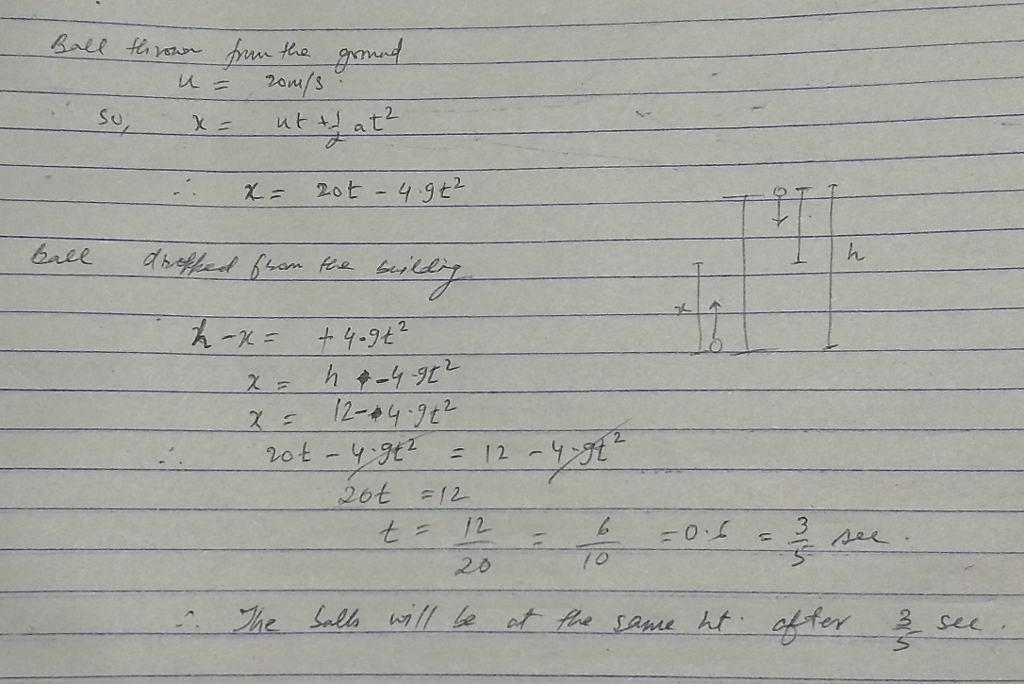Question

A ball is thrown upward from the ground with an initial speed of 20.0 m/s; at the same instant, another ball is dropped from a building 12 m high. After how long will the balls be at the same height?#### Earn Coins

Coins can be redeemed for fabulous gifts.

Similar Homework Help Questions
• ### A ball is thrown upward from the ground with an initial speed of 16.6 m/s; at...

A ball is thrown upward from the ground with an initial speed of 16.6 m/s; at the same instant, another ball is dropped from a building 20 m high. After how long will the balls be at the same height above the ground?

• ### A ball is thrown upward from the ground with an initial speed of 20.2 m/s; at...

A ball is thrown upward from the ground with an initial speed of 20.2 m/s; at the same instant, another ball is dropped from a building 20 m high. After how long will the balls be at the same height?

• ### A ball is thrown upward from the ground with an initial speed of 26.7 m/s; at the same instant, a ball is dropped from a building 13.1 m high

A ball is thrown upward from the ground with an initial speed of 26.7 m/s; at the same instant, a ball is dropped from a building 13.1 m high. After how long will the balls be at the same height?

• ### A ball is thrown upward from the ground with an initial speed of 23.1 m/s; at the same instant, a ball is dropped from a building 15.0 m high

A ball is thrown upward from the ground with an initial speed of 23.1 m/s; at the same instant, a ball is dropped from a building 15.0 m high. After how long will the balls be at the same height?

• ### A ball is thrown upward from the ground with an initial speed of 26.2 m/s; at the same instant a ball is dropped from rest from a building 14 m high

A ball is thrown upward from the ground with an initial speed of 26.2 m/s; at the same instant a ball is dropped from rest from a building 14 m high. After how long will the balls be at the same height?

• ### A ball is thrown upward from the ground with an initial speed of 25 m/s; at the same instant, a ball is dropped from rest from a building 15 m high

A ball is thrown upward from the ground with an initial speed of 25 m/s; at the same instant, a ball is dropped from rest from a building 15 m high. After how long will the balls be at the same height?

• ### A blue ball is thrown upward with an initial speed of 23.9 m/s, from a height...

A blue ball is thrown upward with an initial speed of 23.9 m/s, from a height of 0.5 meters above the ground. 2.9 seconds after the blue ball is thrown, a red ball is thrown down with an initial speed of 7.5 m/s from a height of 31.5 meters above the ground. The force of gravity due to the earth results in the balls each having a constant downward acceleration of 9.81 m/s^2. How long after the blue ball is...

• ### A blue ball is thrown upward with an initial speed of 20 m/s, from a height...

A blue ball is thrown upward with an initial speed of 20 m/s, from a height of 0.8 meters above the ground. 2.4 seconds after the blue ball is thrown, a red ball is thrown down with an initial speed of 5.3 m/s from a height of 22.4 meters above the ground. The force of gravity due to the earth results in the balls each having a constant downward acceleration of 9.81 m/s2. 1) How long after the blue ball...

• ### A blue ball is thrown upward with an initial speed of 24.1 m/s, from a height...

A blue ball is thrown upward with an initial speed of 24.1 m/s, from a height of 0.8 meters above the ground. 2.9 seconds after the blue ball is thrown, a red ball is thrown down with an initial speed of 10.4 m/s from a height of 31.4 meters above the ground. The force of gravity due to the earth results in the balls each having a constant downward acceleration of 9.81 m/s2. What is the height of the red...

• ### A ball is thrown straight up from the ground with an initial velocity of 30.4 m/s; at the same instant, a ball is dropped from the roof of a building 21.1 m high

A ball is thrown straight up from the ground with an initial velocity of 30.4 m/s; at the same instant, a ball is dropped from the roof of a building 21.1 m high. After how long will the balls be at the same height? The acceleration of gravity is 10 m/s2. Answer in units of s